# chen 的个人博客

VX:ZzzChChen
Phone:13403656751
Email:zxydczzs@gmail.com

X

## 尝试一下mysql索引优化！！！

### 最近看了不少的 mysql 调优，其中提到了索引，现在来尝尝鲜。

``````1select * from book_chapter where chapter_name = '第两千五百三十六章 玄雷之命（终章）';
``````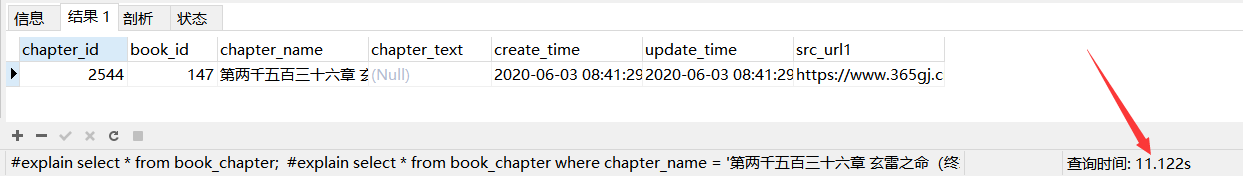#### 这个时候它的执行计划是 ALL，也就是全表扫描。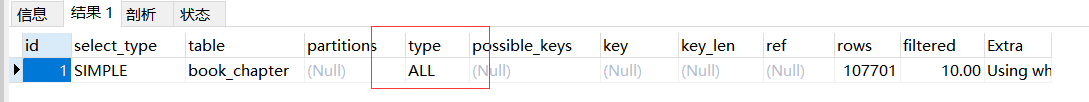``````1alter table book_chapter add index chapter_name(chapter_name);
``````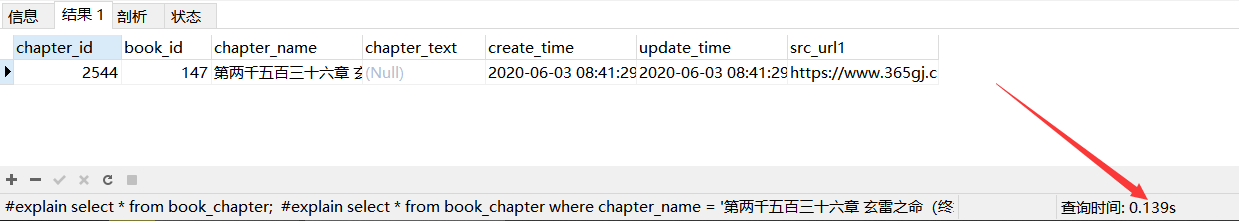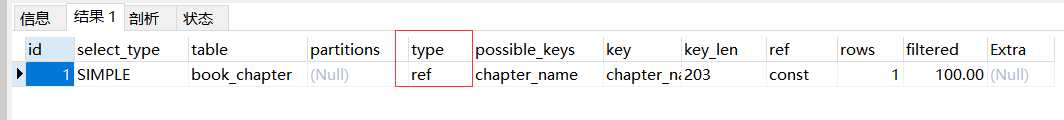ref 的解释：不使用唯一索引，而是使用普通索引或者唯一性索引的部分前缀，索引要和某个值相比较，可能会找到多个符合条件的行

``````1select chapter_id, book_id,chapter_name from book_chapter where chapter_name = '第两千五百三十六章 玄雷之命（终章）';
``````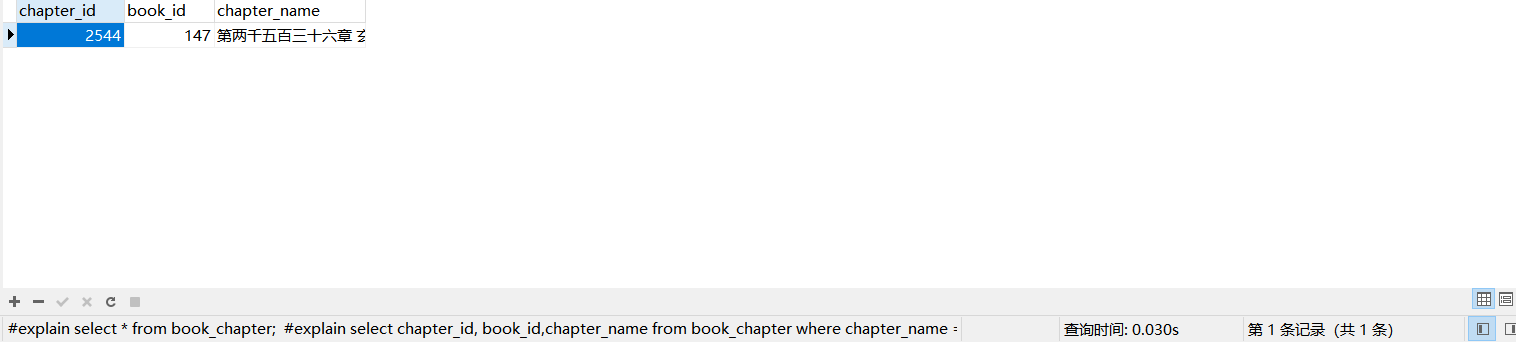## 以上均是自己理解，有不对之处请在下方留言指出来，虚心接受指教? ? ? ****

`````` 1-- 精准查询chapter_name，不带索引，时间较长，11.122s;
2SELECT * FROM book_chapter WHERE chapter_name = '第两千五百三十六章 玄雷之命（终章）';
3
4-- 执行计划，type显示ALL，全表扫描;
5EXPLAIN SELECT * FROM book_chapter WHERE chapter_name = '第两千五百三十六章 玄雷之命（终章）';
6
7-- 创建索引，索引名称chapter_name，被索引的字段chapter_name;
8ALTER TABLE book_chapter ADD INDEX chapter_name(chapter_name);
9
10-- 创建索引后精准查询chapter_name，时间变短，0.139s;
11SELECT * FROM book_chapter WHERE chapter_name = '第两千五百三十六章 玄雷之命（终章）';
12
13-- 创建索引后执行计划,type显示ref,不使用唯一索引，而是使用普通索引或者唯一性索引的部分前缀，索引要和某个值相比较，可能会找到多个符合条件的行;
14EXPLAIN SELECT * FROM book_chapter WHERE chapter_name = '第两千五百三十六章 玄雷之命（终章）';
15
16-- 带查询字段的语句，时间更短，0.030s;
17SELECT chapter_id, book_id, chapter_name FROM book_chapter WHERE chapter_name = '第两千五百三十六章 玄雷之命（终章）';
18
19-- 创建索引并且带查询字段的执行计划
20EXPLAIN SELECT chapter_id, book_id, chapter_name FROM book_chapter WHERE chapter_name = '第两千五百三十六章 玄雷之命（终章）';
21
22-- 删除索引;
23DROP INDEX chapter_name ON book_chapter;
``````